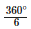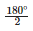• +91 9971497814
• info@interviewmaterial.com

# RD Chapter 10- Congruent Triangles Ex-VSAQS Interview Questions Answers

### Related Subjects

Question 1 : Define complementary - angles.

Answer 1 : Two angles whose sum is 90°, are called complementary angles.

Question 2 : Define supplementary- angles.

Answer 2 : Two angles whose sum is 180°, are called supplementary angles.

Question 3 : Define adjacent - angles.

Answer 3 : Two angles which have common vertex and one arm common are called adjacent angles.

Question 4 : The complement of an acute angles is…….

Answer 4 : The complement of an acute angles is an acute angle.

Question 5 : The supplement of an acute angles is………

Answer 5 : The supplement of an acute angles is a obtuse angle.

Question 6 : The supplement of a right angle is…….

Answer 6 : The supplement of a right angle is a right angle.

Question 7 : Write the complement of an angle of measure x°.

Answer 7 : The complement of x° is (90° – x)°

Question 8 : Write the supplement of an angle of measure 2y°.

Answer 8 : The supplement of 2y° is (180° – 2y)°

Question 9 : If a wheel has six spokes equally spaced, then find the measure of the angle between two adjacent spokes.

Total measure of angle around a point = 360°
Number of spokes = 6
∴ Angle between the two adjacent spokes == 60°

Question 10 : An angle is equal to its supplement. Determine its measure.

Let required angle = x°
Then its supplement angle = 180° – x
x = 180° – x
⇒ x + x = 180°
⇒  2x = 180° ⇒  x == 90°
∴ Required angle = 90°

Todays Deals### RD Chapter 10- Congruent Triangles Ex-VSAQS Contributorskrishan

Name:
Email:

# Latest News# 9000 interview questions in different categories